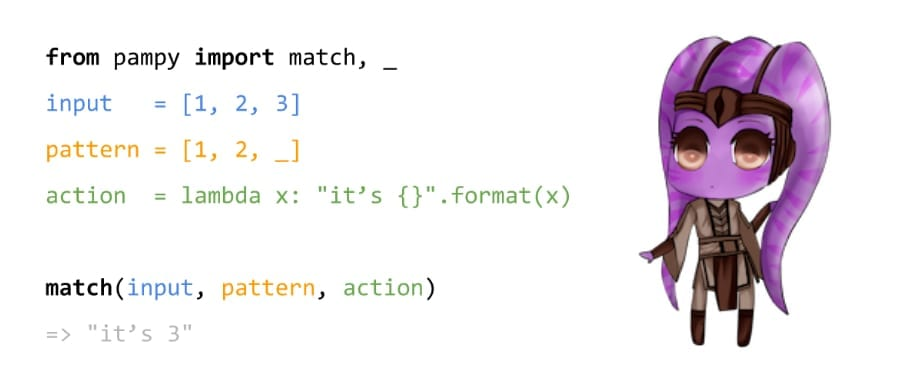# Python 强大的模式匹配工具—Pampy

## 1.安装

`pip install pampy`

## 2.使用

```from pampy import match, HEAD, TAIL, _

x = [-1, -2, -3, 0, 1, 2, 3]

print(match(x, [-1, TAIL], lambda t: [-1, tuple(t)]))
# => [-1, (-2, -3, 0, 1, 2, 3)] ```

```from pampy import match, HEAD, TAIL, _

x = [-1, -2, -3, 0, 1, 2, 3]

print(match(x, [HEAD, _, _, 0, TAIL], lambda h, a, b, t: (set([h, a, b]), tuple(t))))
# => ({-3, -1, -2}, (1, 2, 3)) ```

```from pampy import match, HEAD, TAIL, _
my_dict = {
'global_setting': [1, 3, 3],
'user_setting': {
'face': ['beautiful', 'ugly'],
'mind': ['smart', 'stupid']
}
}
result = match(my_dict, { _: {'face': _}}, lambda key, son_value: (key, son_value))
print(result)
# => ('user_setting', ['beautiful', 'ugly']) ```

```import re
from pampy import match, HEAD, TAIL, _
def what_is(pet):
return match(pet,
re.compile('(\w+)，(\w)\w+鳕鱼\$'),     lambda mygod, you: you + "像鳕鱼",
)

print(what_is('我的天，你长得真像鳕鱼'))     # => '你像鳕鱼' ```

​Python实用宝典 (pythondict.com)